# The Journal of Accounting and Management, Vol 6, No 2 (2016)

Instructions for the preparation of the two-page digest

Journal of Accounting and Management JAM vol. 6, no. 2(2016)

An Equilibrium Model for the Romanian Economy

Cătălin Angelo IOAN1, Gina IOAN2

Abstract: The model presented in this article is an adaptation of the IS-LM model for an open economy in which both the static aspects and dynamic ones are approached. The determination of marginal main indicators of GDP and interest rates, allow to identify problems and the directions of action to achieve economic equilibrium.

Keywords: equilibrium, GDP, investments, interest rate, consumption

1 Introduction

The economic equilibrium problem, has origins and manifestations lost in the mists of time.

Economic thinkers from different current and ideologies as François Quesnay, Léon Walras, Vilfredo Pareto, Alfred Marshall studied this problem.

In the XX century, John Maynard Keynes formulate a first economic equilibrium model for a closed economy without governmental sector.

Because the equilibrium problem bore controversies on economics, it get further researches, today being analyzed the fluctuations that accompany this process.

Within theory of economic equilibrium, a synthetic analysis it is the IS-LM model consisting of simultaneous equilibrium in two markets, money market and the goods and services in an autarkic economy.

Starting from Keynesian macroeconomic equilibrium, in 1937, Roy Harrod, James Meade and John Hicks tried to express mathematical majors relations of Keynes' theory (Hahn, F.H., 1977).

Subsequent developments of Alvin Hansen of 1949 and 1953 play an important role in systematizing known IS-LM model, in his book (Hansen A.H., 1959) in order to get the curve IS, Hansen calls the investment demand function of Keynes and the neoclassical paradigm and for the LL curve is the curve of points where supply and demand (Beaud M., Dostaler G., 1996).

The IS-LM model (King R. G., 1993; Lawn P. A., 2003; Martínez-García E., Vilán D., 2012; Romer D., 2000; Schmitt-Grohe S., Uribe M., 2002; Weerapana, A., 2003) was the basis for further researches – theoretical or empirical.

After Samuelson and Solow which include the original model of the Phillips curve (1960), Fleming Mundell and Fleming include balance of payments (1960 and 1962).

Also, Modigliani and Friedman use the consumption function (1954 and 1957) and Tobin includes the demand for money (1958).

Although economic literature that explores New Open Economy Macroeconomics (NOEM models) is not as rich as that of the closed economy model, it is a significant theoretical edifice for the current macroeconomic modeling: Bergin (Bergin P., 2004), Schmitt-Grohe and Uribe (Smith, R.P.; Zoega, G., 2009), Justiniano and Preston (Justiniano A., Preston B., 2008), (Justiniano A., Preston B., 2010), Martínez-García and Vilán (Martínez-García E., Vilán D., 2012).

The new approach enables researchers to explain the new changes that have occurred in the international macroeconomic environment.

In this paper we propose, based on ideological vision and studies of the most important researchers in the field to determine a model for an open economy, with applications on the Romanian case.

2 The model equations

The first equation of the model is the formula of the aggregate demand:

1. D(t)=C(t)+G(t)+I(t)+EX(t)-IM(t)

where

• D(t) – the aggregate demand at the moment t;

• C(t) – the actual final consumption of households at the moment t;

• G(t) – the actual final consumption of the government at the moment t;

• I(t) – the investments at the moment t;

• EX(t) – the exports at the moment t;

• IM(t) – the imports at the moment t

A second equation relates the actual final consumption of households according to disposable income:

1. C(t)=cVDI(t)+C0, C0R, cV0

where

• DI(t) – the disposable income at the moment t;

• cV – the marginal propensity to consume, cV=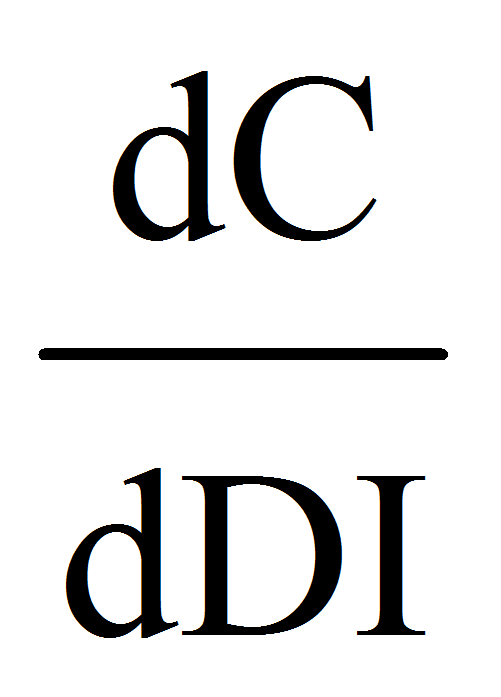0;

• C0 is the intrinsic achieved autonomous consumption of households

1. G(t)=iGTI(t), iG(0,1)

where

• TI(t) – the total income at the moment t;

• iG – the marginal index of final consumption of the government according to total income

1. TI(t)=TR(t)+OR(t)

where:

• TR(t) – tax rate at the moment t;

• OR(t) – other revenues at the moment t

1. OR(t)=iORY(t)+OR0, iOR(0,1), OR0R

where:

• Y(t) – the output at the moment t;

• iOR – the marginal index of other revenues according to the output;

• OR0 – the autonomous other revenues

1. I(t)=iYY(t)+irr(t), iY(0,1), ir0

where:

• I(t) – investments at the moment t;

• r(t) – the real interest rate at the moment t;

• iY – the rate of investments;

• ir – a factor of influence on the investment rate

1. IM(t)=imYY(t)+ceiCH(t)+IM0, imY0, cei0, IM0R

where:

• CH(t) – the exchange rate of the national currency based on the euro at the moment t;

• imY – the rate of imports;

• cei – a factor of imports influence on the exchange rate

• IM0 – the autonomous imports

1. EX(t)=exYY(t)+ceeCH(t)+EX0, exY0, cee0, EX0R

where:

• exY – the rate of exports;

• cee – a factor of exports influence on the exchange rate

• EX0 – the autonomous exports

1. CH(t)=rCHt+CH0, rCH, CH0R

where:

• rCH – the marginal index of the exchange rate according to time;

• CH0 – the intercept of the regression

1. TF(t)=cTFY(t)+TF0, cTF(0,1), TF0R

where:

• TF(t) – the government transfers at the moment t;

• cTF – the marginal index of government transfers according to the output;

• TF0 – the autonomous government transfers

1. TR(t)=tYY(t)+TR0, tY(0,1), TR0R

where:

• tY – the marginal index of tax rate according to the output;

• TR0 – the intercept of the regression

1. DI(t)=Y(t)+TF(t)-TR(t)

2. D(t)=Y(t) – the equation of equilibrium at the moment t

3. MD(t)=mdYY(t)+mdrr(t), mdY(0,1), mdr0

where:

• MD(t) – the money demand in the economy at the moment t;

• mdY – the rate of money demand in the economy;

• mdr – a factor of influencing the demand for currency from the interest rate

1. MS(t)=mSt+M0, mM,M0R

where:

• MS(t) – the money supply in the economy at the moment t;

• mS – the marginal index of the money supply according to time;

• M0 – the intercept of the regression

MD(t)=MS(t) – the equation of equilibrium at the moment t.

3 The equilibrium at a fixed moment

From (4), (5), (11) we get:

1. TI(t)=(tY+iOR)Y(t)+TR0+OR0

From (3), (17):

1. G(t)=(iGtY+iGiOR)Y(t)+iG(TR0+OR0)

From (7), (9):

1. IM(t)=imYY(t)+ceirCHt+ceiCH0+IM0

From (8), (9):

1. EX(t)=exYY(t)+ceerCHt+ceeCH0+EX0

From (10), (11), (12) we get:

1. DI(t)=(1+cTF-tY)Y(t)+TF0-TR0

From (2), (21):

1. C(t)=(cV+cVcTF-cVtY)Y(t)+cV(TF0-TR0)+C0

Now, from (1), (6), (18), (19), (20), (22) we have:

1. D(t)=(cV+cVcTF-cVtY+iGtY+iGiOR+iY+exY-imY)Y(t)+irr(t)+(ceerCH-ceirCH)t+

cV(TF0-TR0)+iG(TR0+OR0)+(cee-cei)CH0+C0+EX0-IM0

From (13) and (23) we get the first equation of the equilibrium:

1. (cV+cVcTF-cVtY+iGtY+iGiOR+iY+exY-imY-1)Y(t)+irr(t)+(ceerCH-ceirCH)t+

cV(TF0-TR0)+iG(TR0+OR0)+(cee-cei)CH0+C0+EX0-IM0=0

and from (14), (15), (16) we get the second equation of the equilibrium

1. mdYY(t)+mdrr(t)-mSt-M0=0

Let note now:

1. =cV+cVcTF-cVtY+iGtY+iGiOR+iY+exY-imY-1

=ceirCH-ceerCH

=cV(TF0-TR0)+iG(TR0+OR0)+(cee-cei)CH0+C0+EX0-IM0

The equilibrium equations become:

1.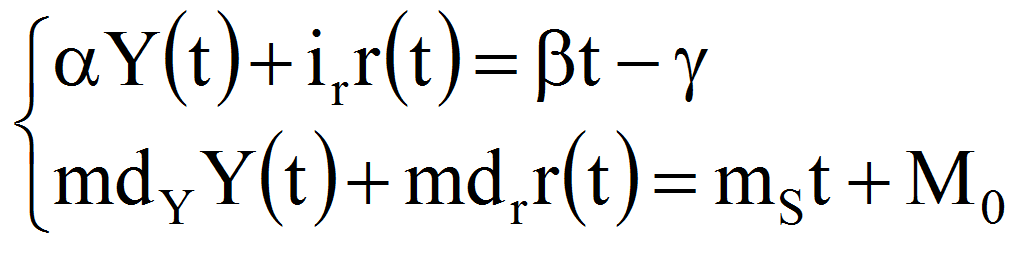The solutions of equilibrium are (noted with same symbols without being a confusion):

1.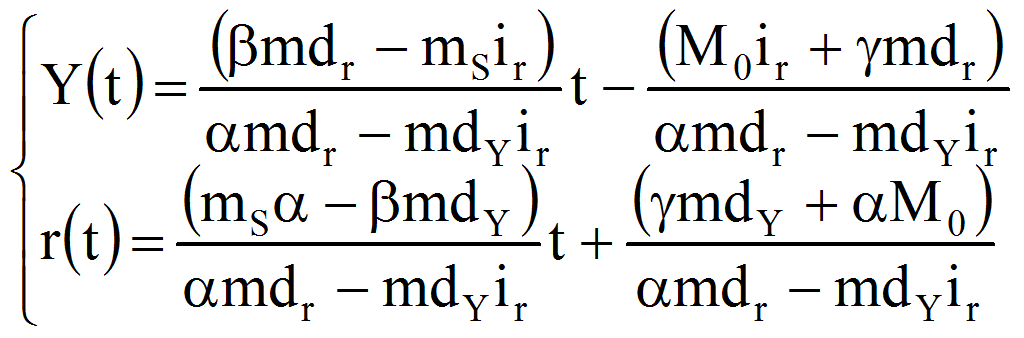At equilibrium, replacing (28) in (1)-(16), we have:

1.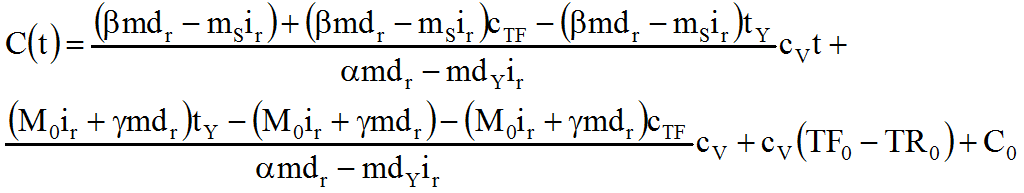2. G(t)=iGTI(t)=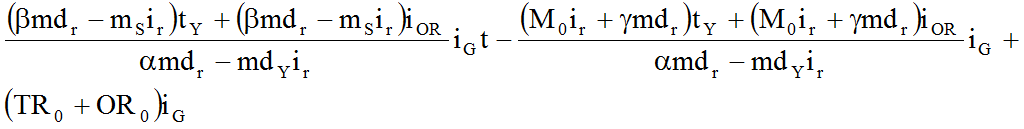1. TI(t)=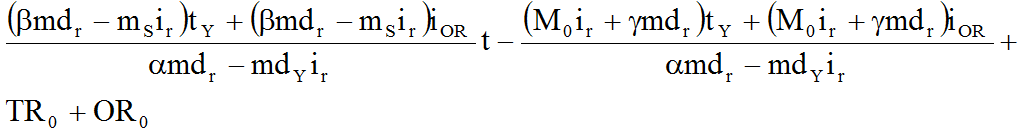2. OR(t)=iORY(t)+OR0=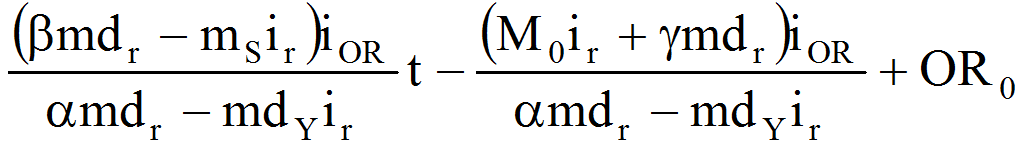3. I(t)=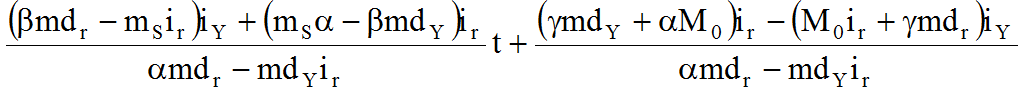4. IM(t)=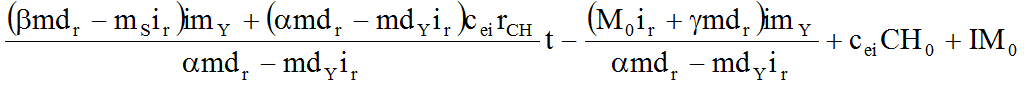5. EX(t)=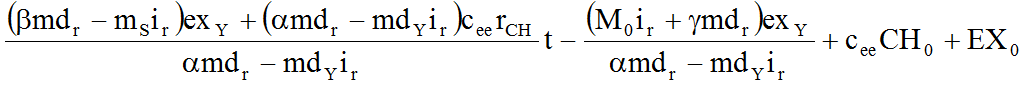6. CH(t)=rCHt+CH0

7. TF(t)=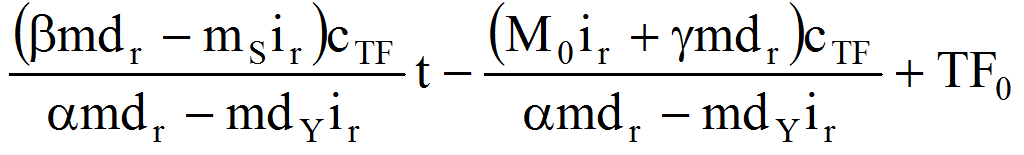8. TR(t)=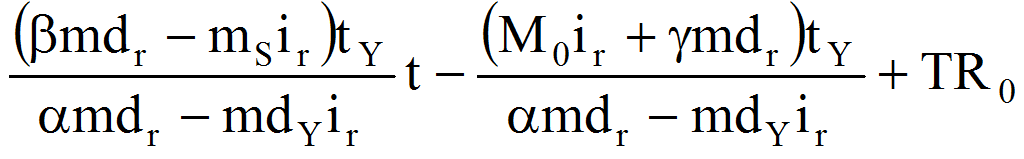9.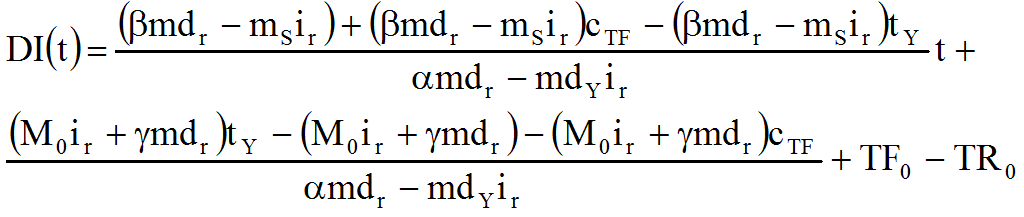MD(t)=MS(t)=mSt+M0.

4 The variations of equilibrium output and real interest rate based on the parameter values

First of all, we will compute the derivatives of functions , and in function of the parameters of the model.

1.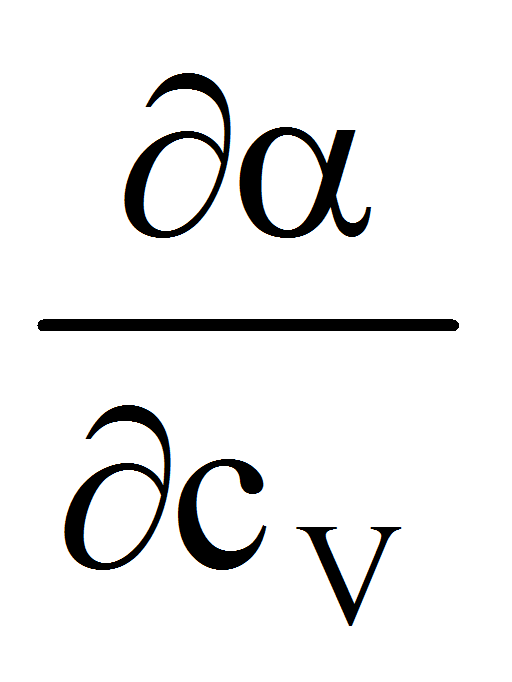=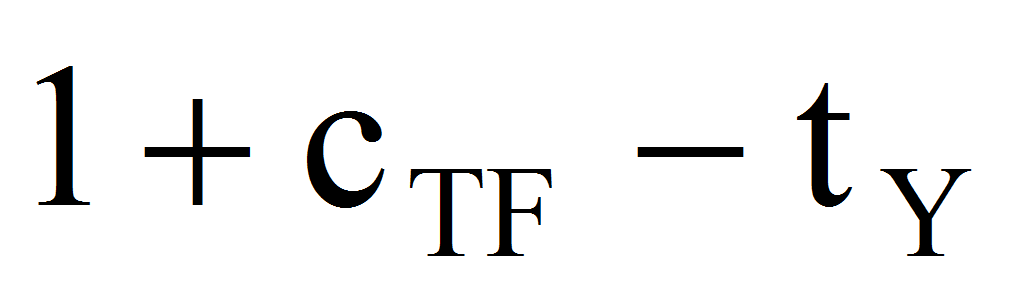,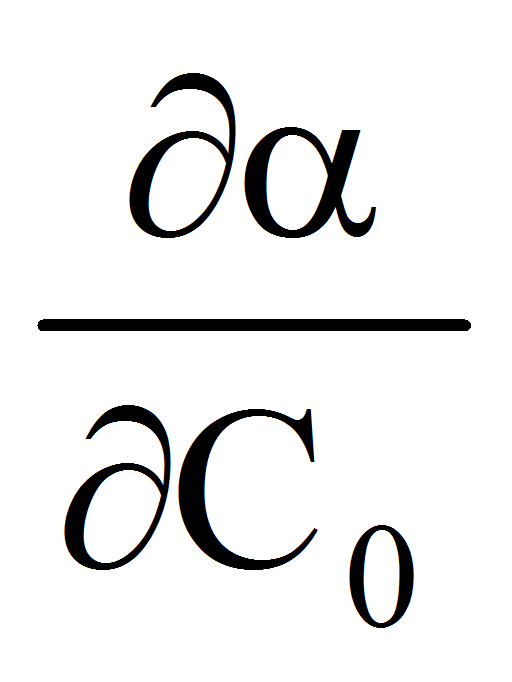=0,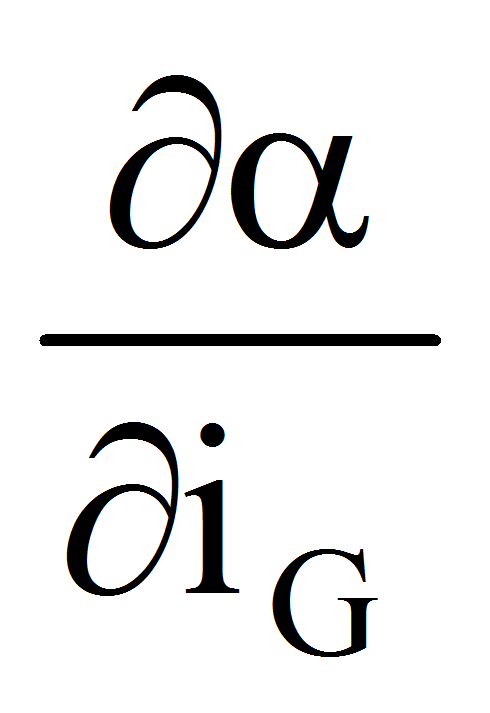=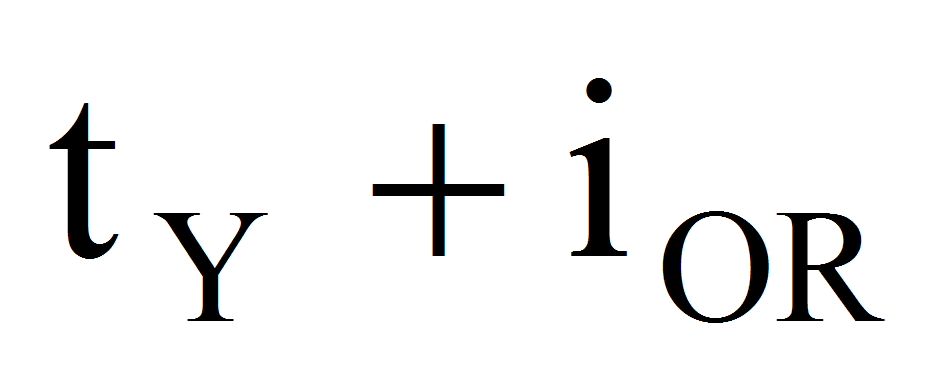,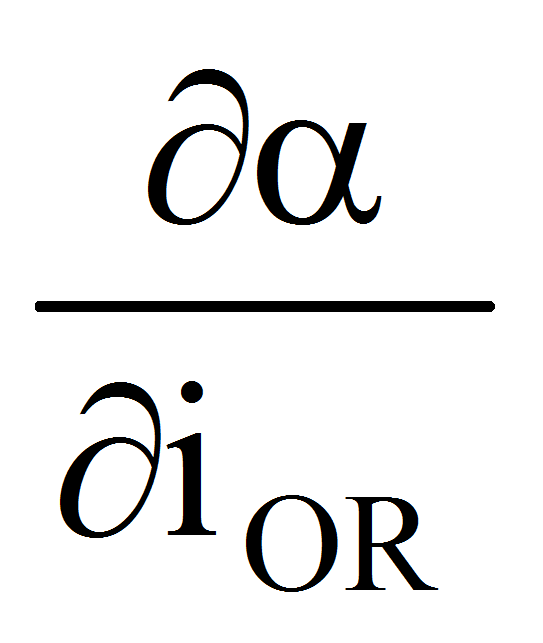=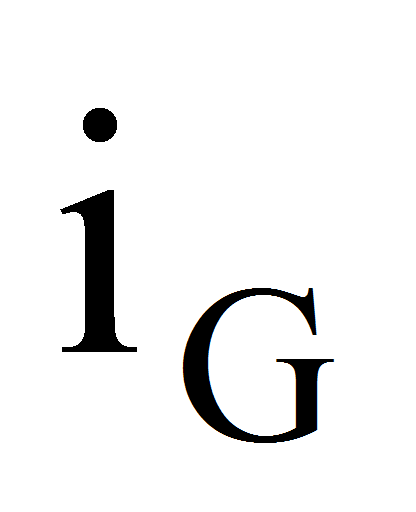,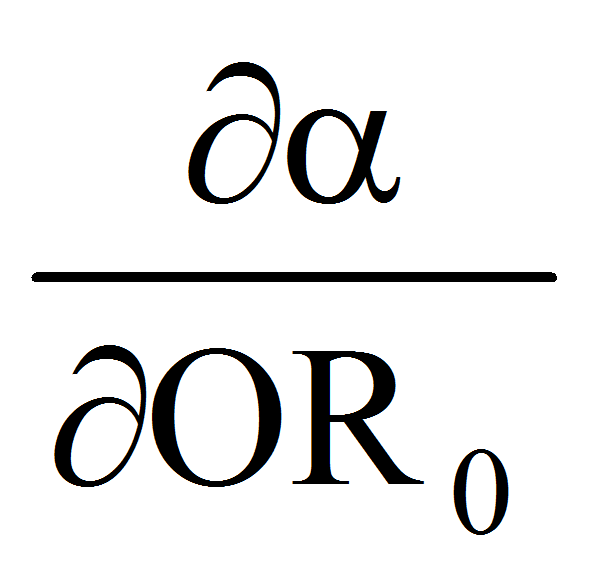=0,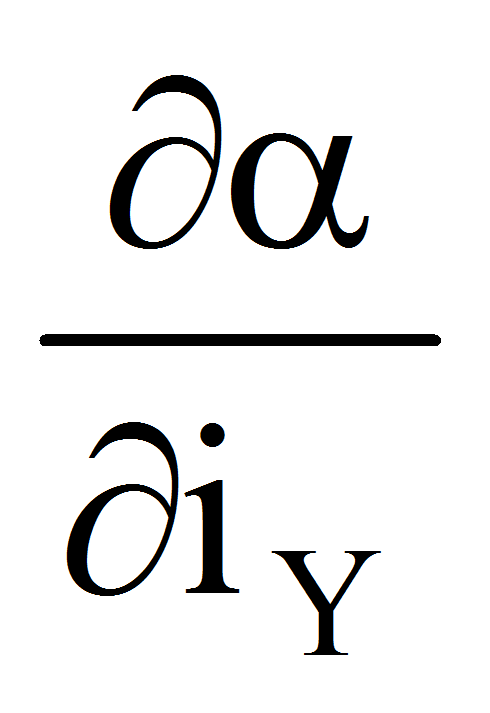=1,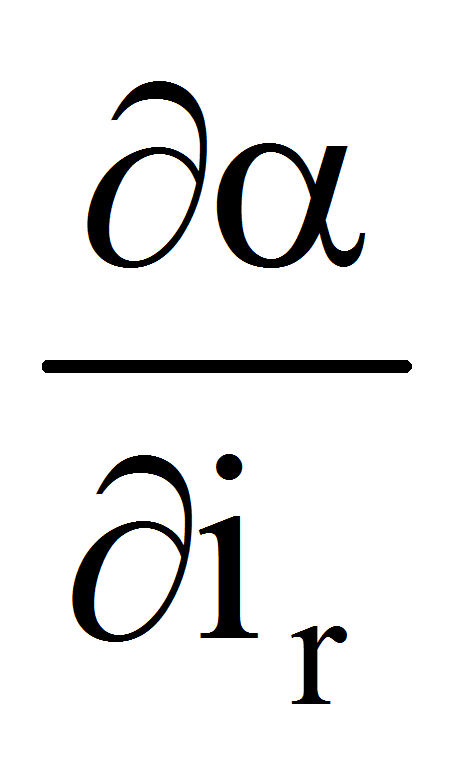=0,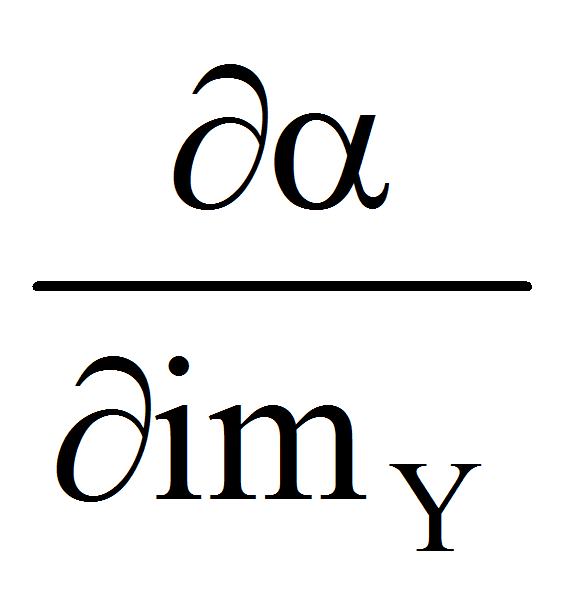=-1,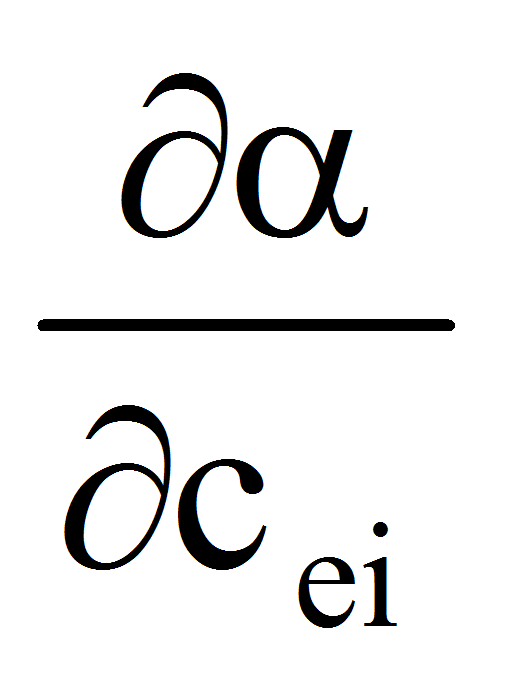=0,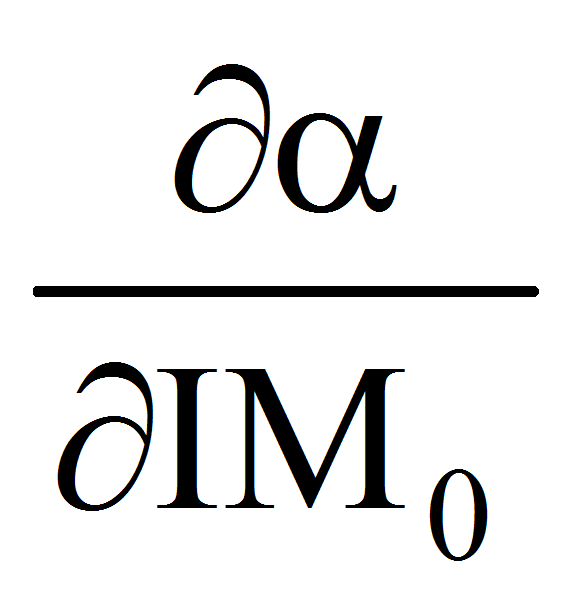=0,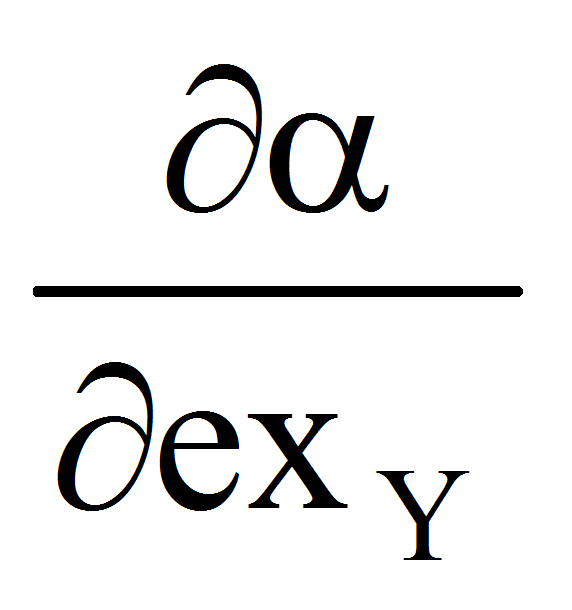=1,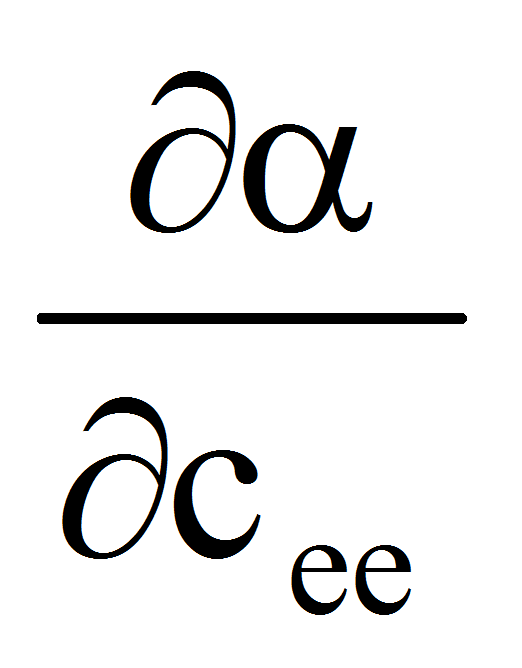=0,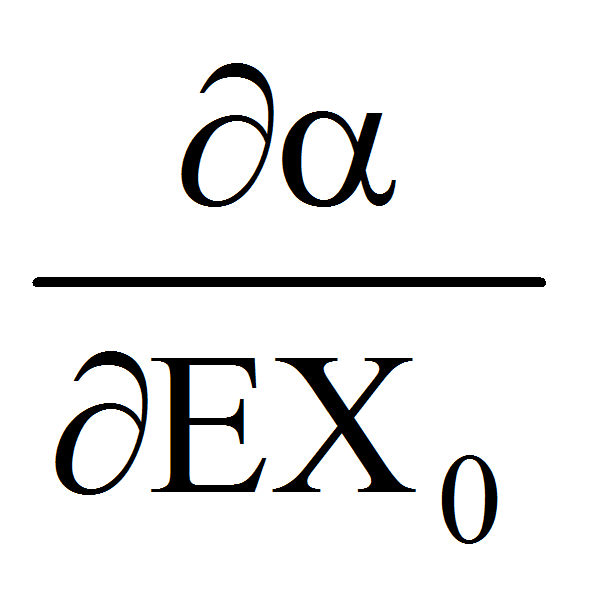=0,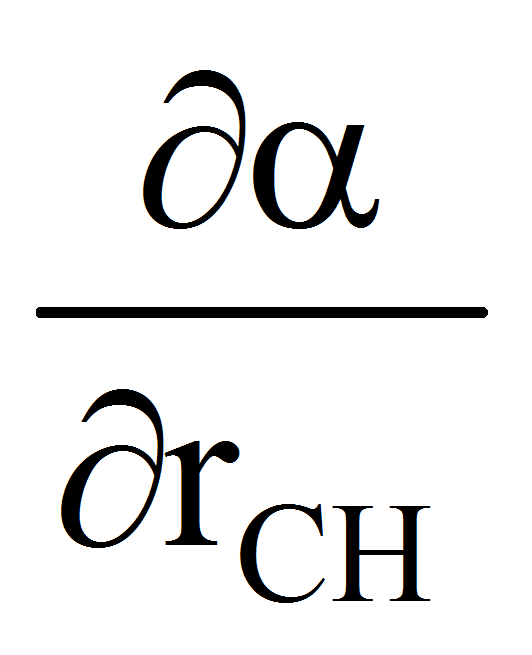=0,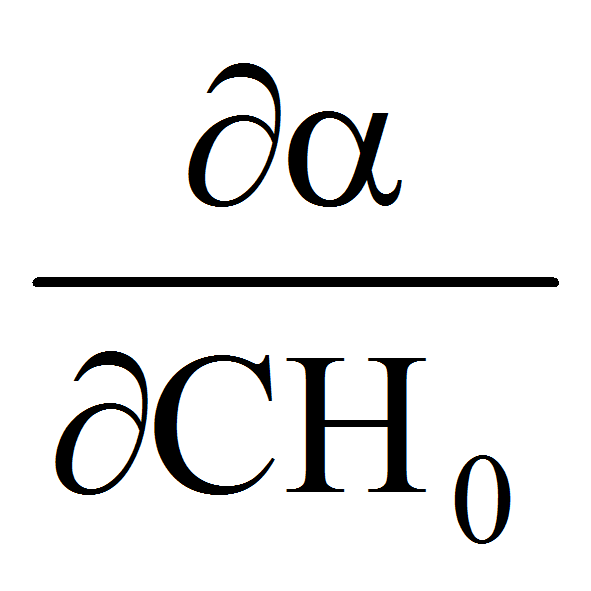=0,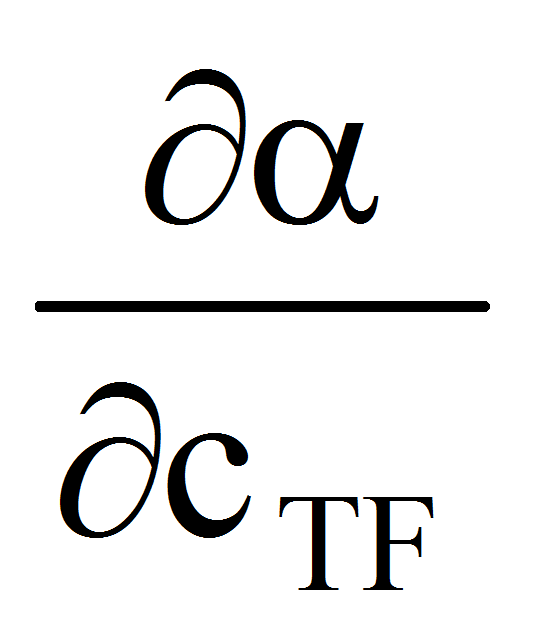=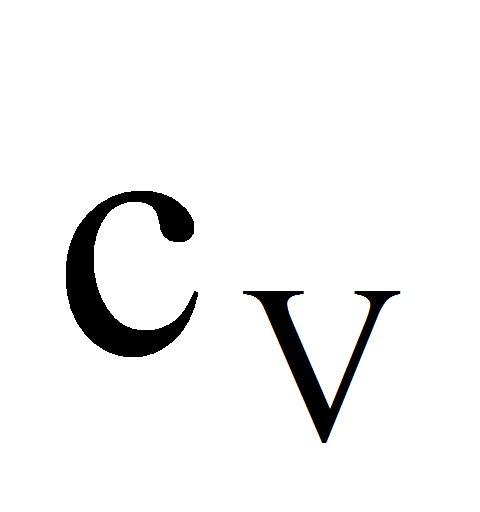,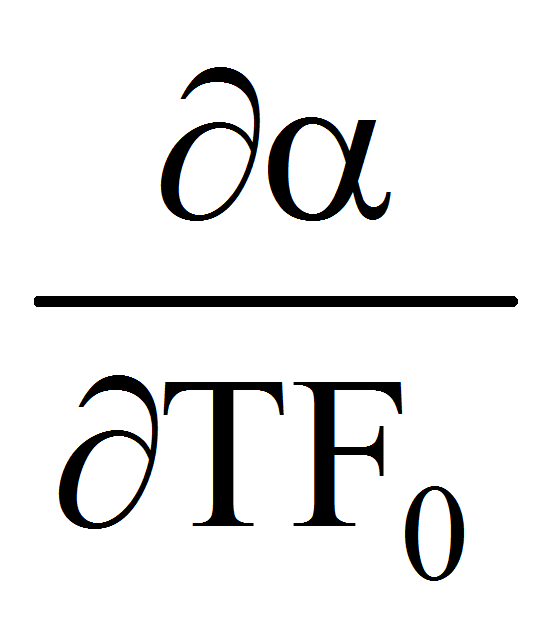=0,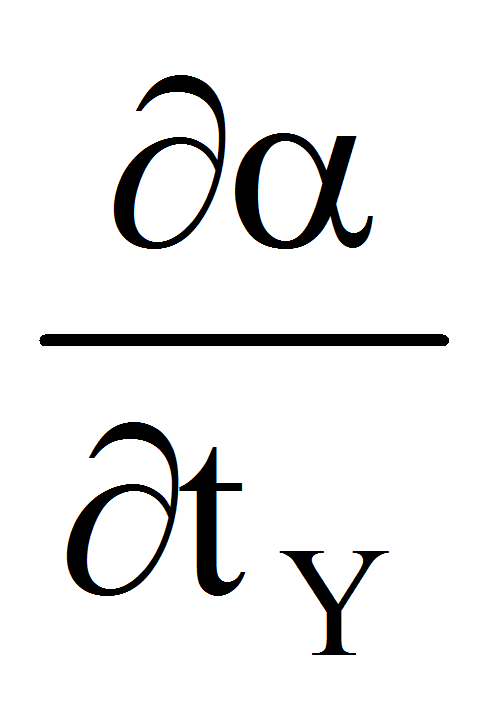=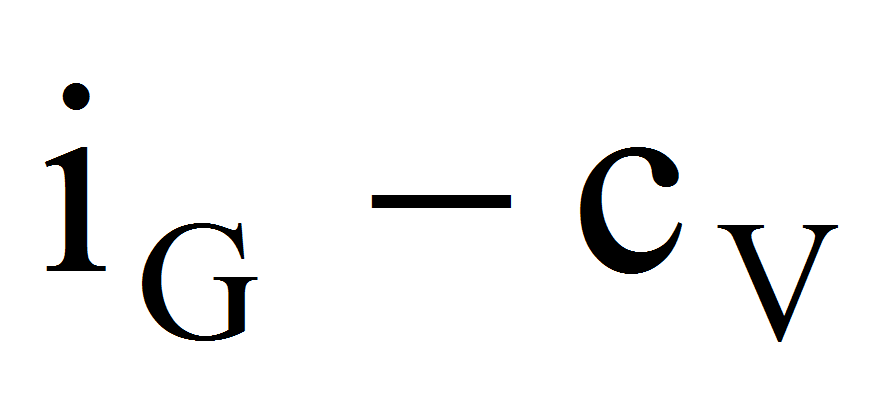,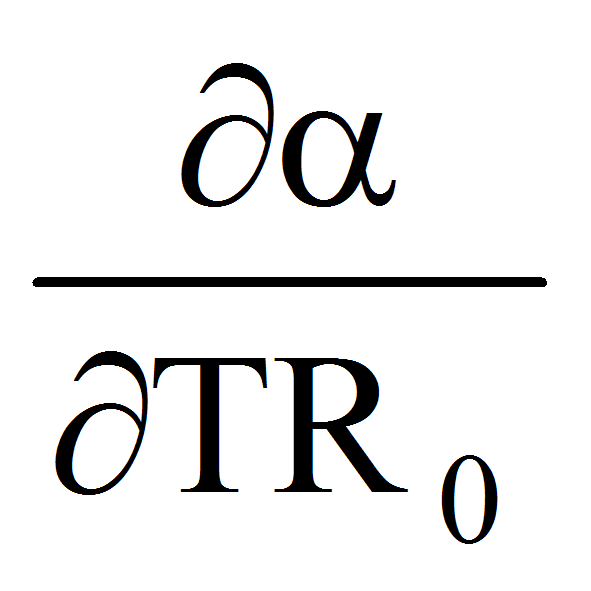=0,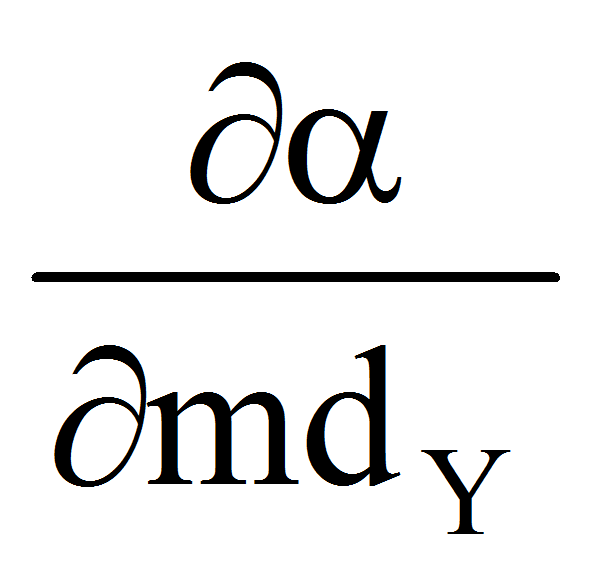=0,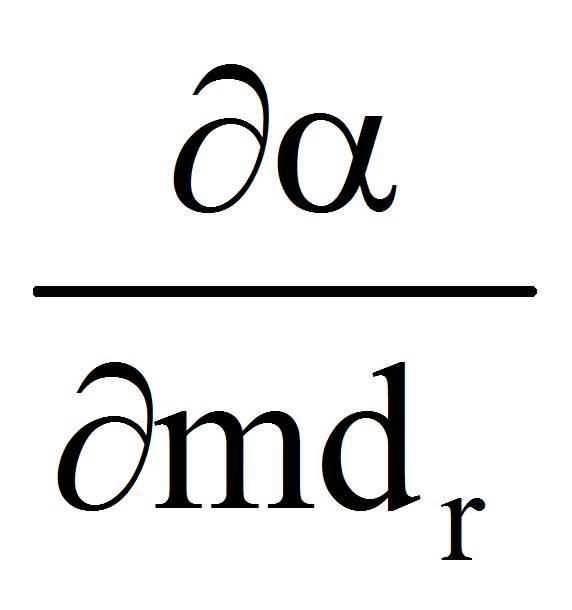=0,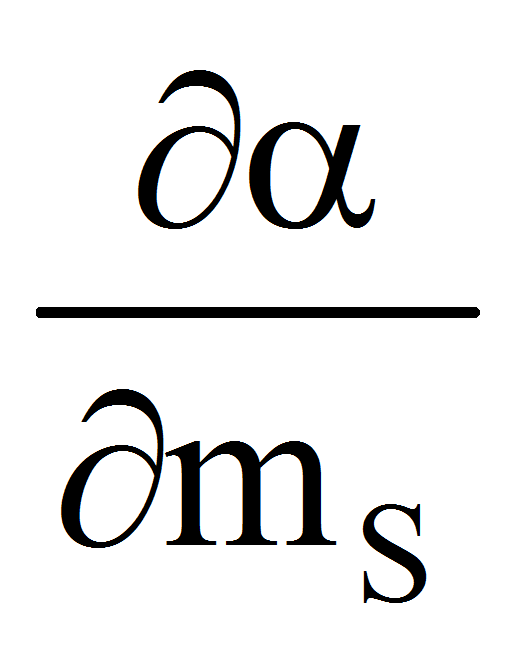=0,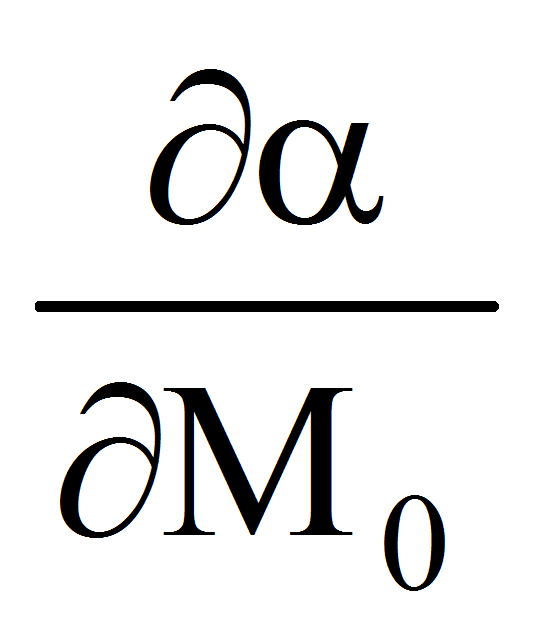=0

2.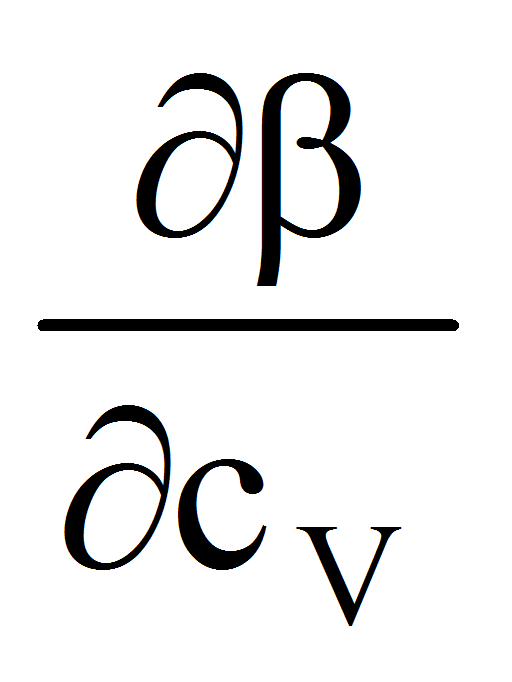=0,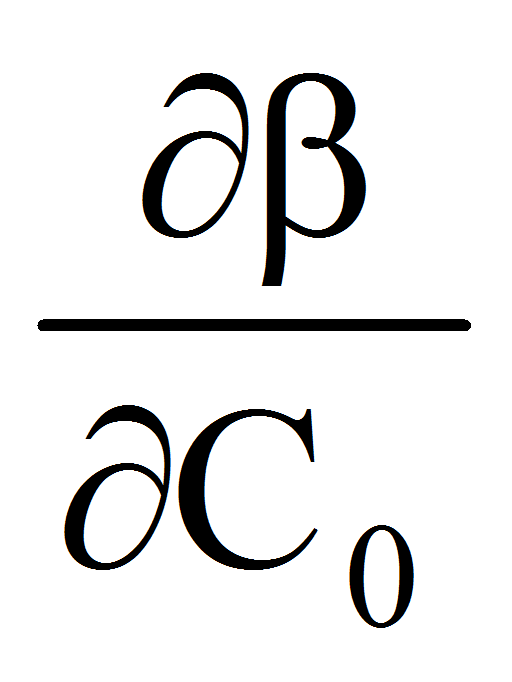=0,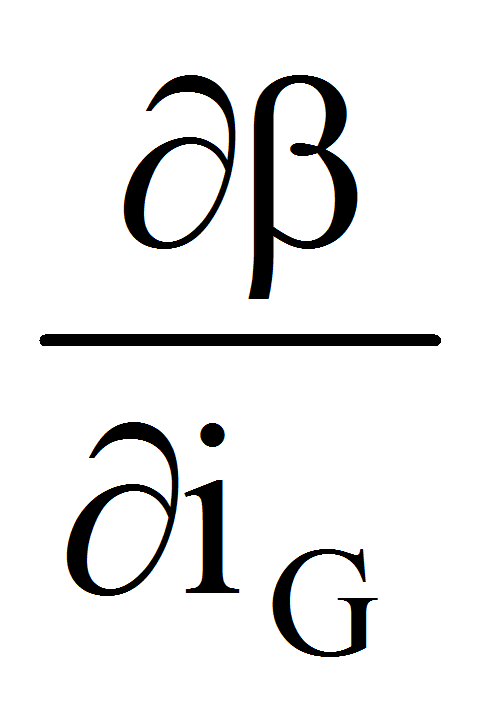=0,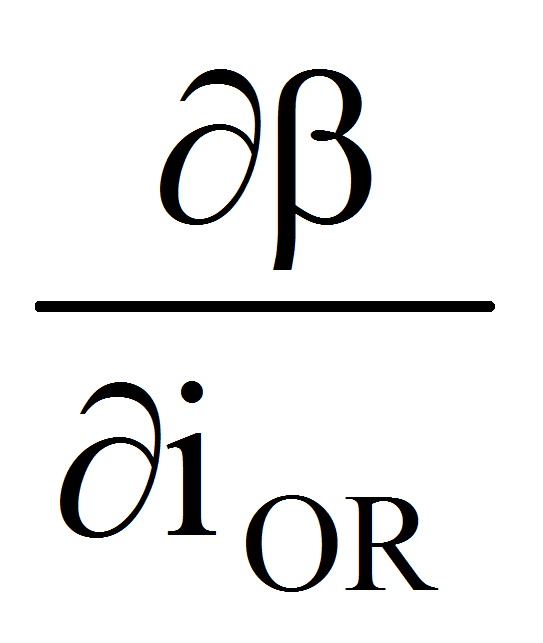=0,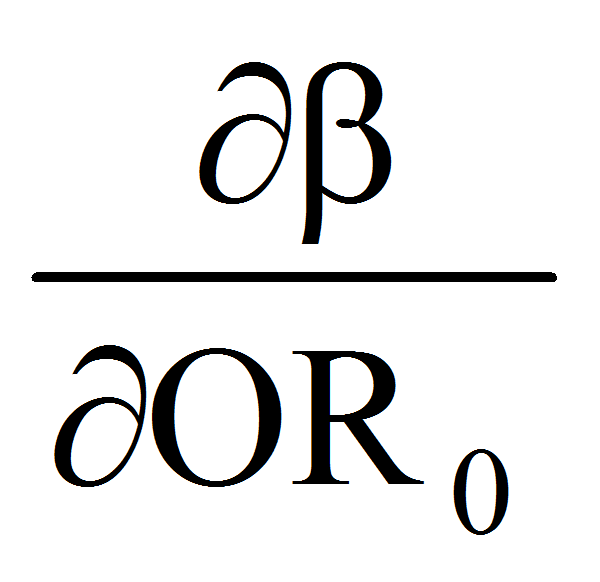=0,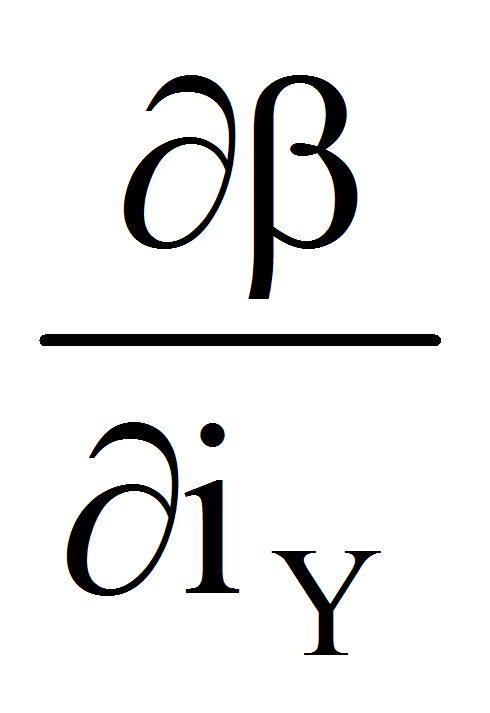=0,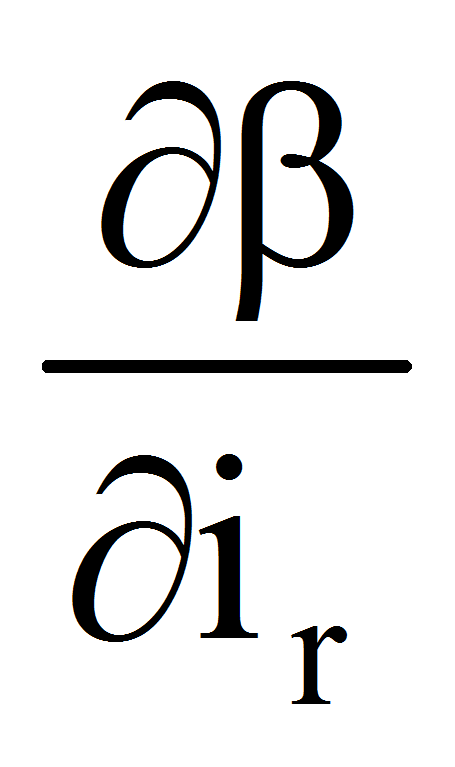=0,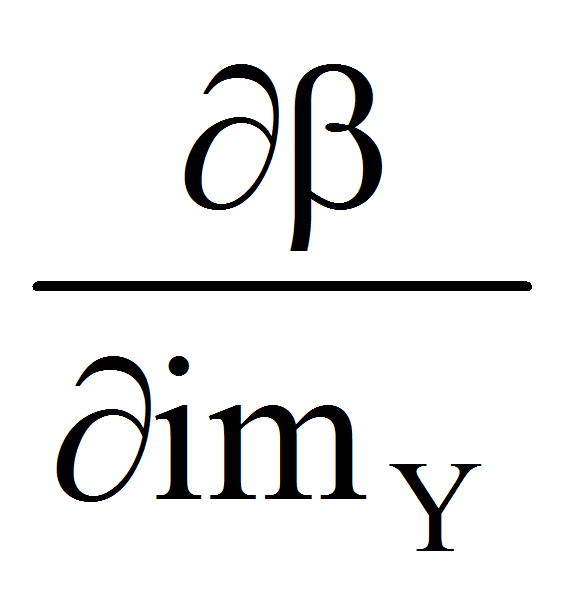=0,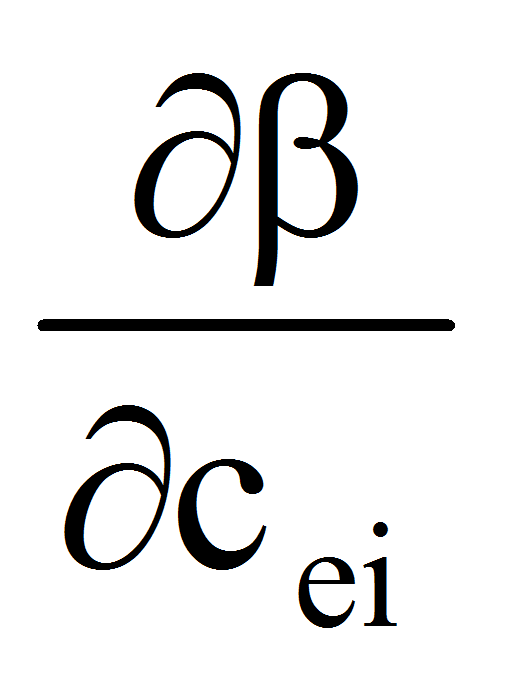=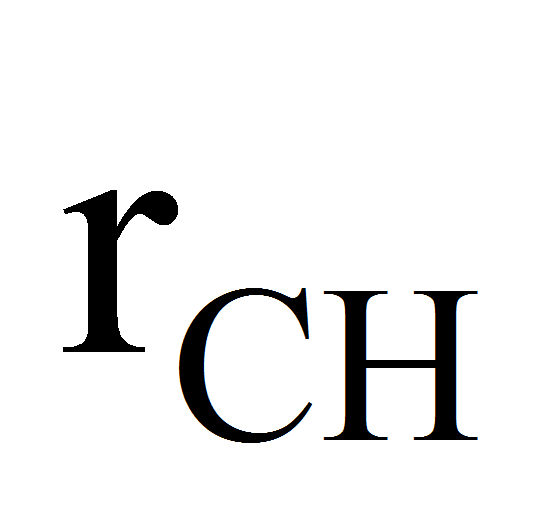,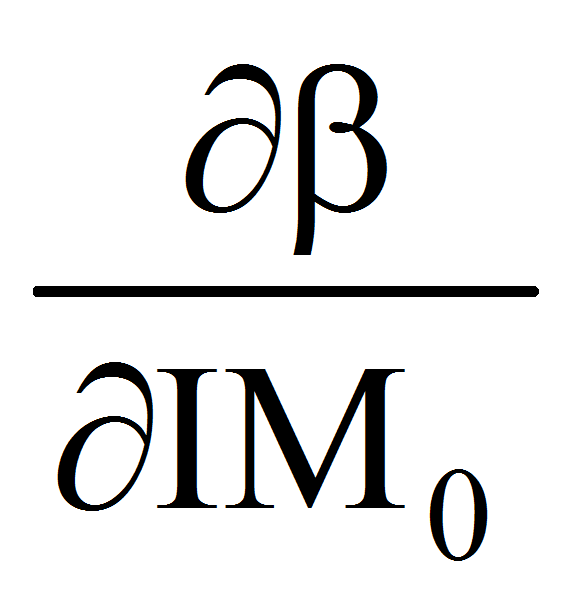=0,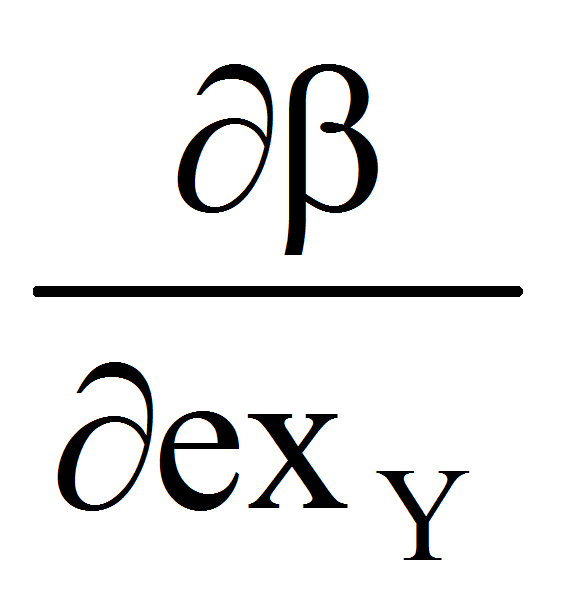=0,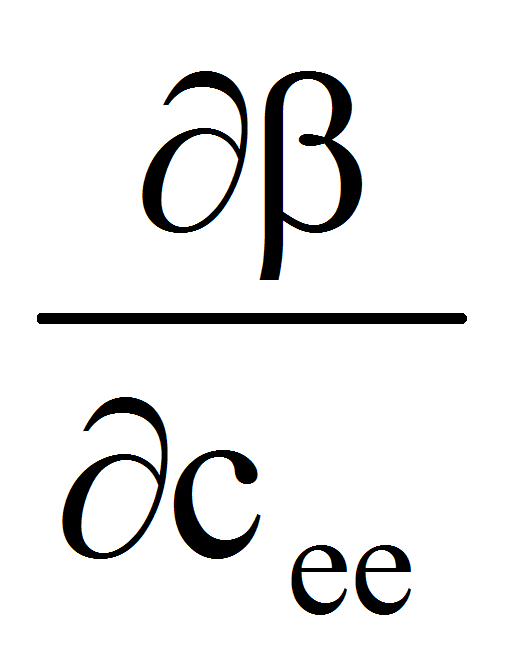=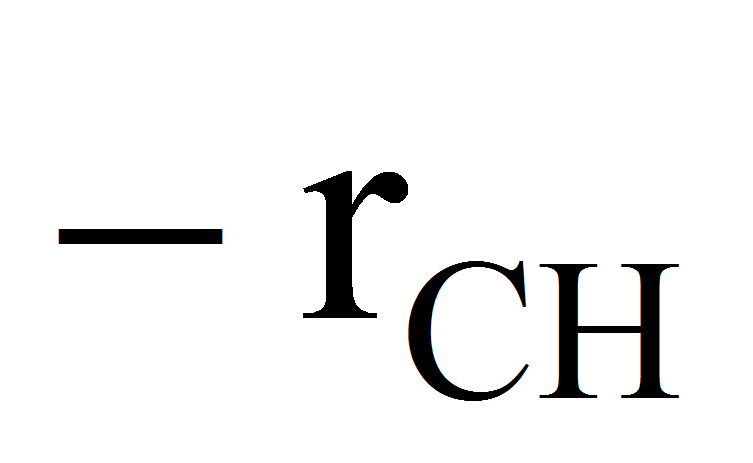,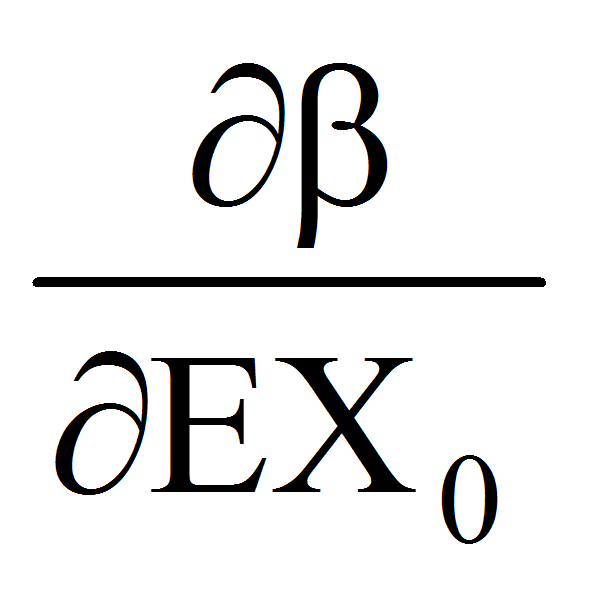=0,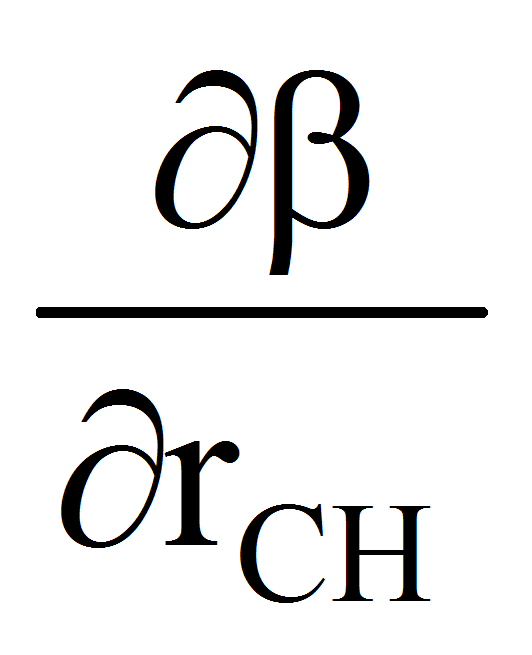=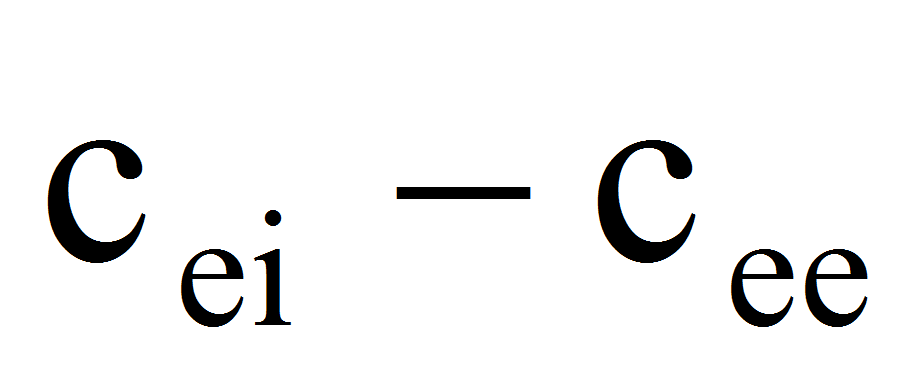,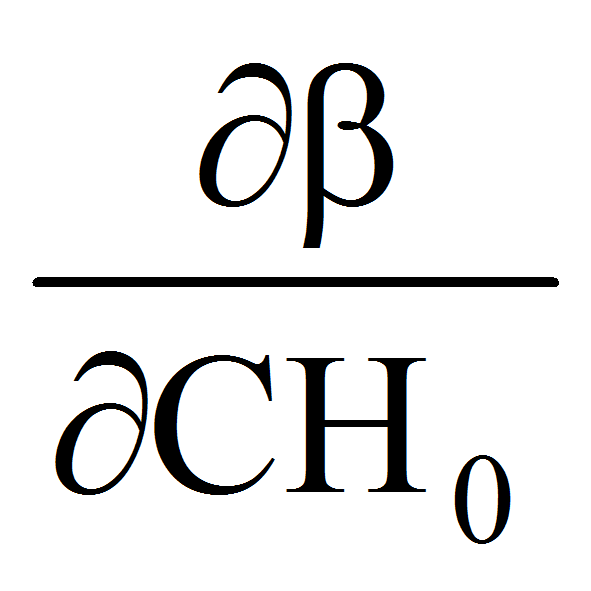=0,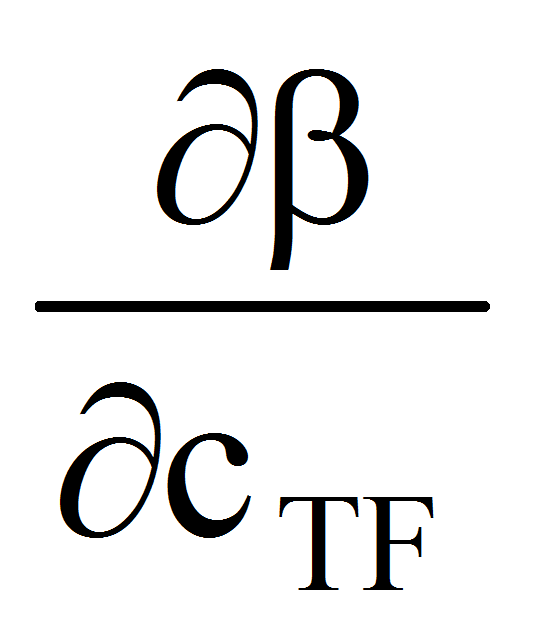=0,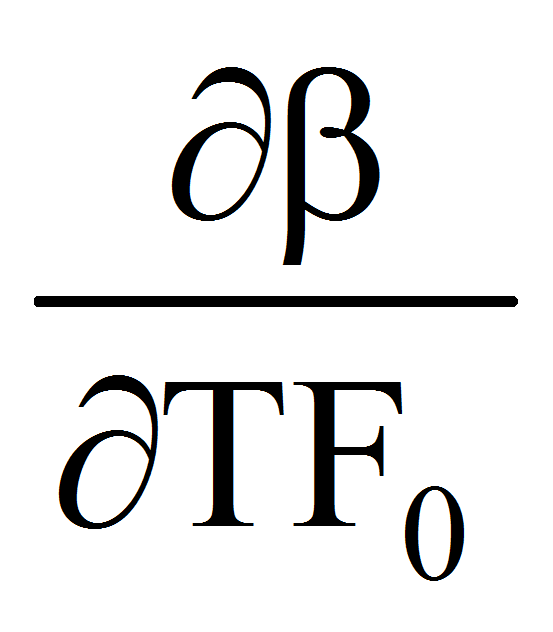=0,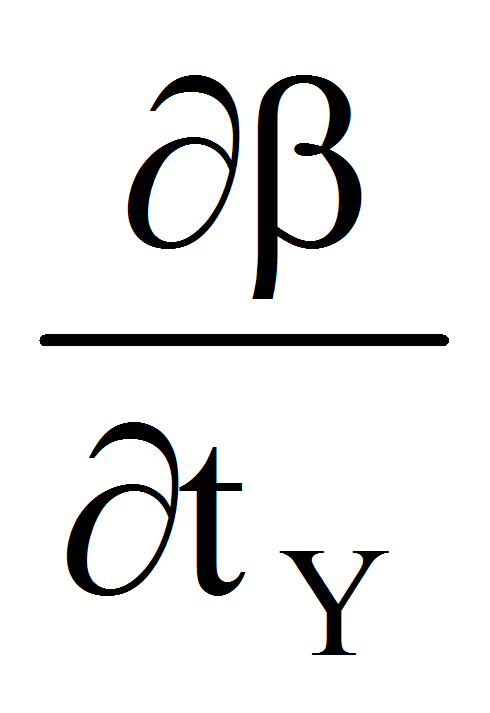=0,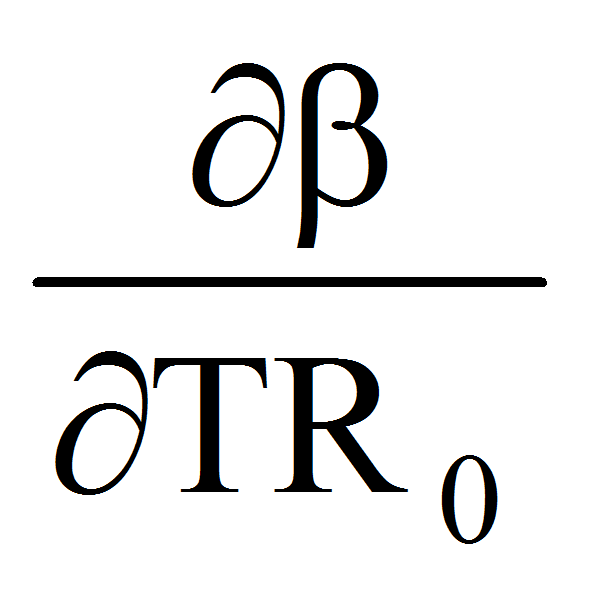=0,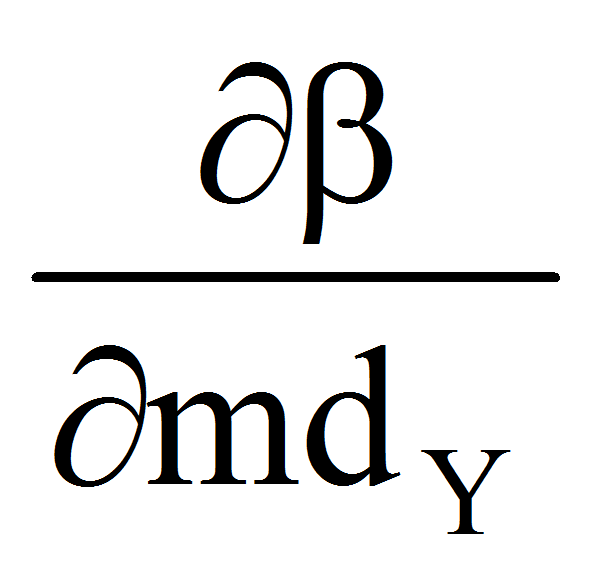=0,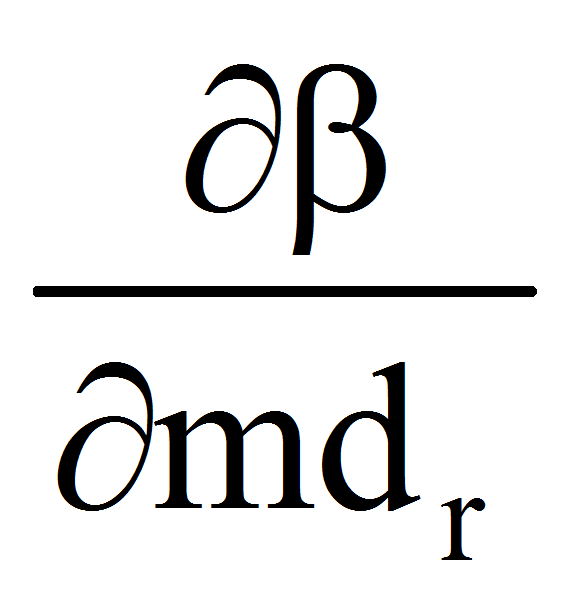=0,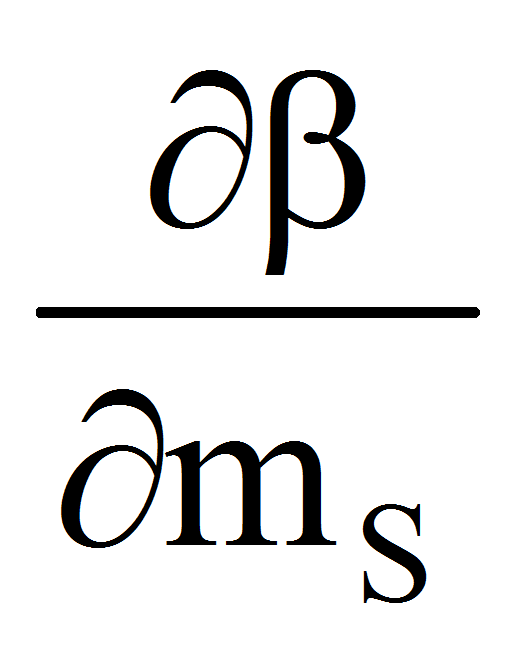=0,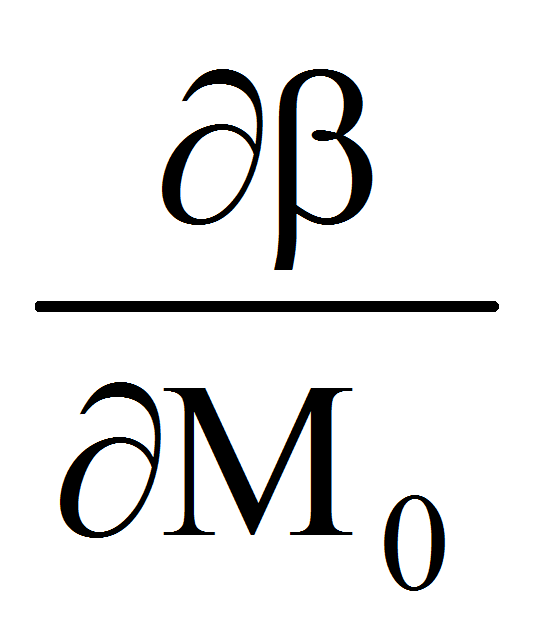=0

3.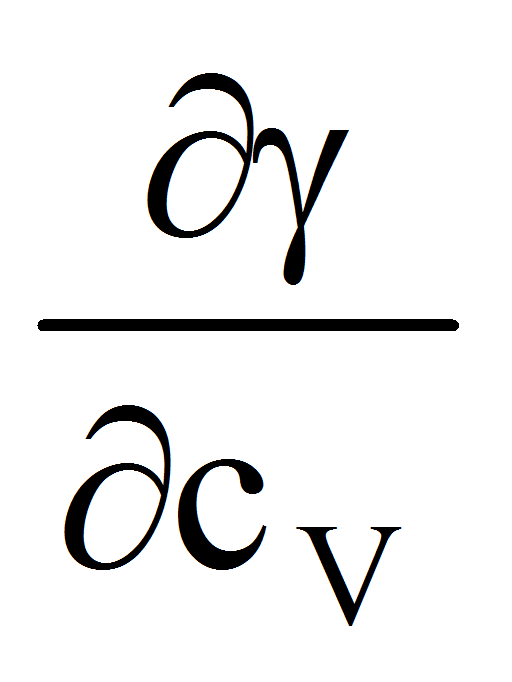=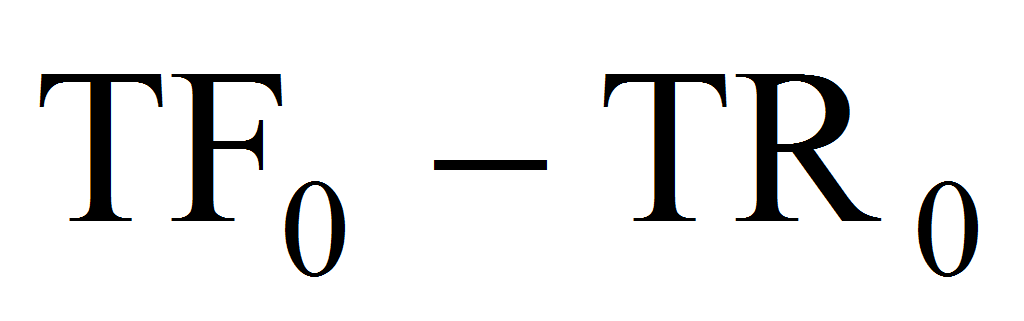,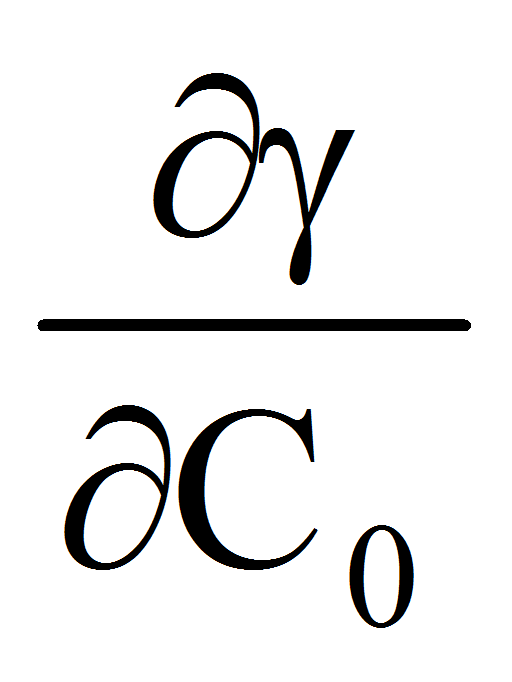=1,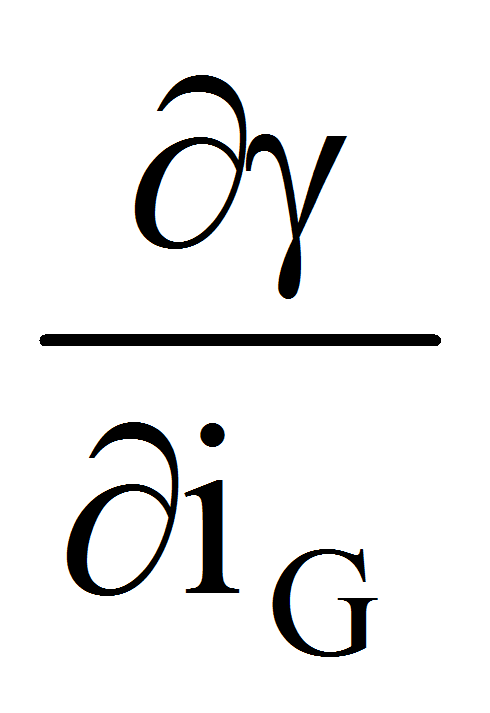=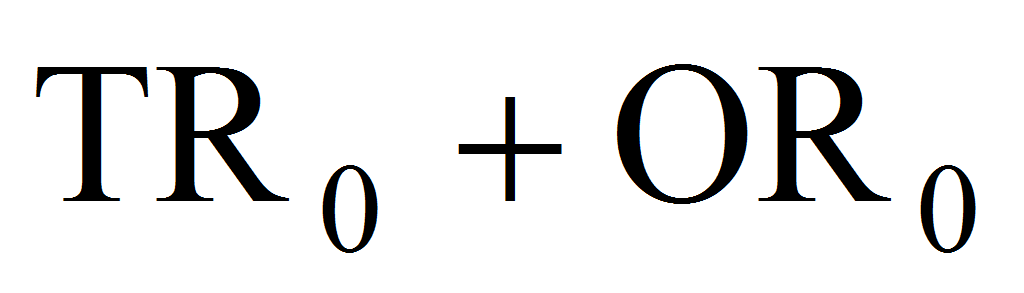,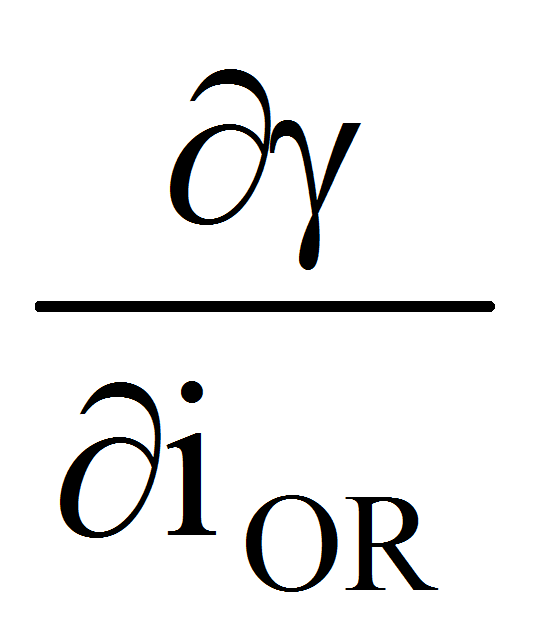=0,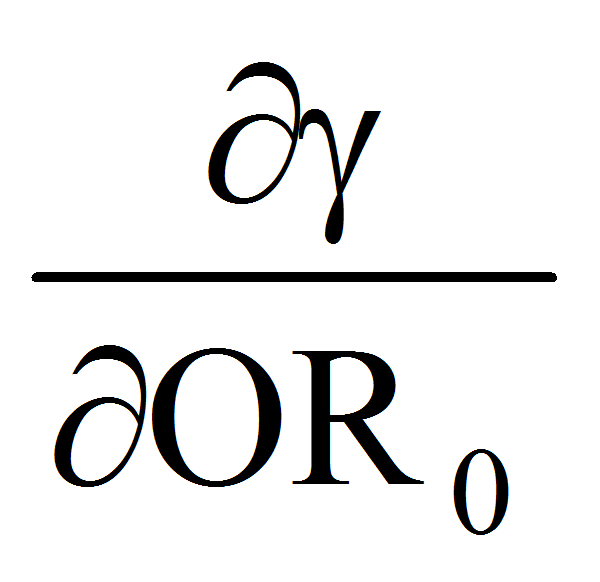=,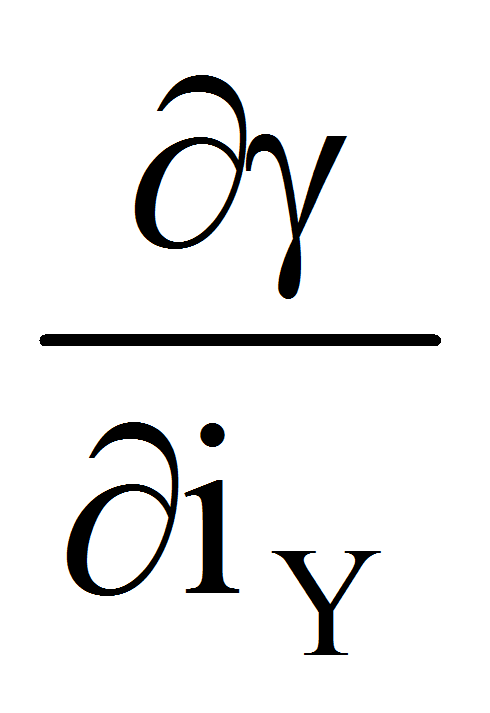=0,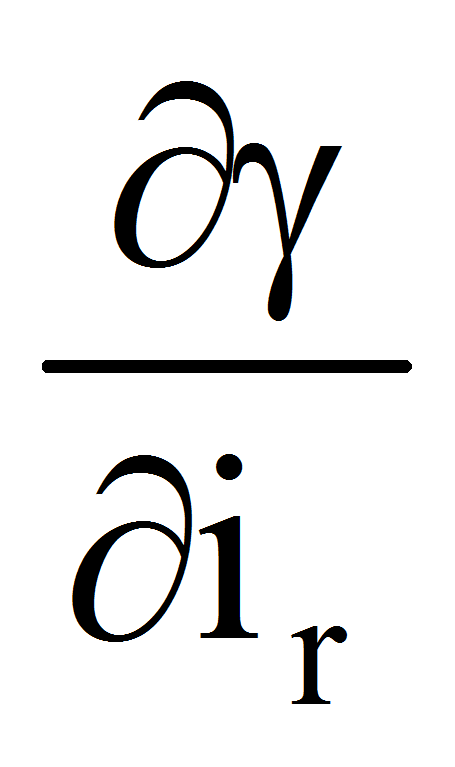=0,